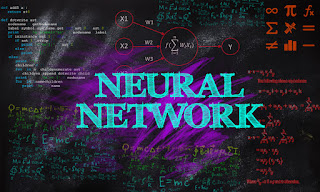# Learn Neural Network Didactic Course in Online with Scratch Examples

## About Neural Network:

This full course video on Neural Network tutorial will help you understand what a neural network is, how it works, and what are the different types of neural networks. You will learn how each neuron processes data, what are activation functions, and how a neuron fires. You will get an idea about backpropagation and gradient descent algorithms. You will have a look at the convolution neural network and how it identifies objects in an image. Finally, you will understand about the recurrent neural networks and lstm in detail.### Course Timings:

1. Animated Video  00:52

2. What Is A Neural Network  06:35

3. What is Deep Learning  07:40
4. What is Artificial Neural Network  09:00
5. How Does Neural Network Works  10:37
6. Advantages of Neural Network 13:39
7. Applications of Neural Network  14:59
8. Future of Neural Network 17:03
9. How Does Neural Network Works  19:10
10. Types of Artificial Neural Network  29:27
11. Use Case-Problem Statement 34:57
12. Use Case-Implementation  36:17
13. Backpropagation & Gradient Descent  01:06:00
14. Loss Function  01:10:26
15. Gradient Descent 01:11:26
16. Backpropagation  01:13:07
17. Convolutional Neural Network 01:17:54
18. How Image recognition Works 01:17:58
19. Introduction to CNN  01:20:25
20. What is Convolutional Neural Network  01:20:51
21. How CNN recognize Images  01:25:34
22. Layers in Convolutional Neural Network  01:26:19
23. Use Case implementation using CNN  01:39:21
24. What is a Neural Network  02:21:24
25. Popular Neural Network  02:23:08
26. Why Recurrent Neural Network  02:24:19
27. Applications of Recurrent Neural Network  02:25:32
28. how does an RNN works 02:28:42
29. vanishing And Exploding Gradient Problem  02:31:02
30. Long short term memory  02:35:54
31. use case implementation of LSTM  02:44:32

Copyrights: Simplilearn

Learn Mailchimp Didactic full course in online: Click here

To Get Udemy Courses For Free: Click here